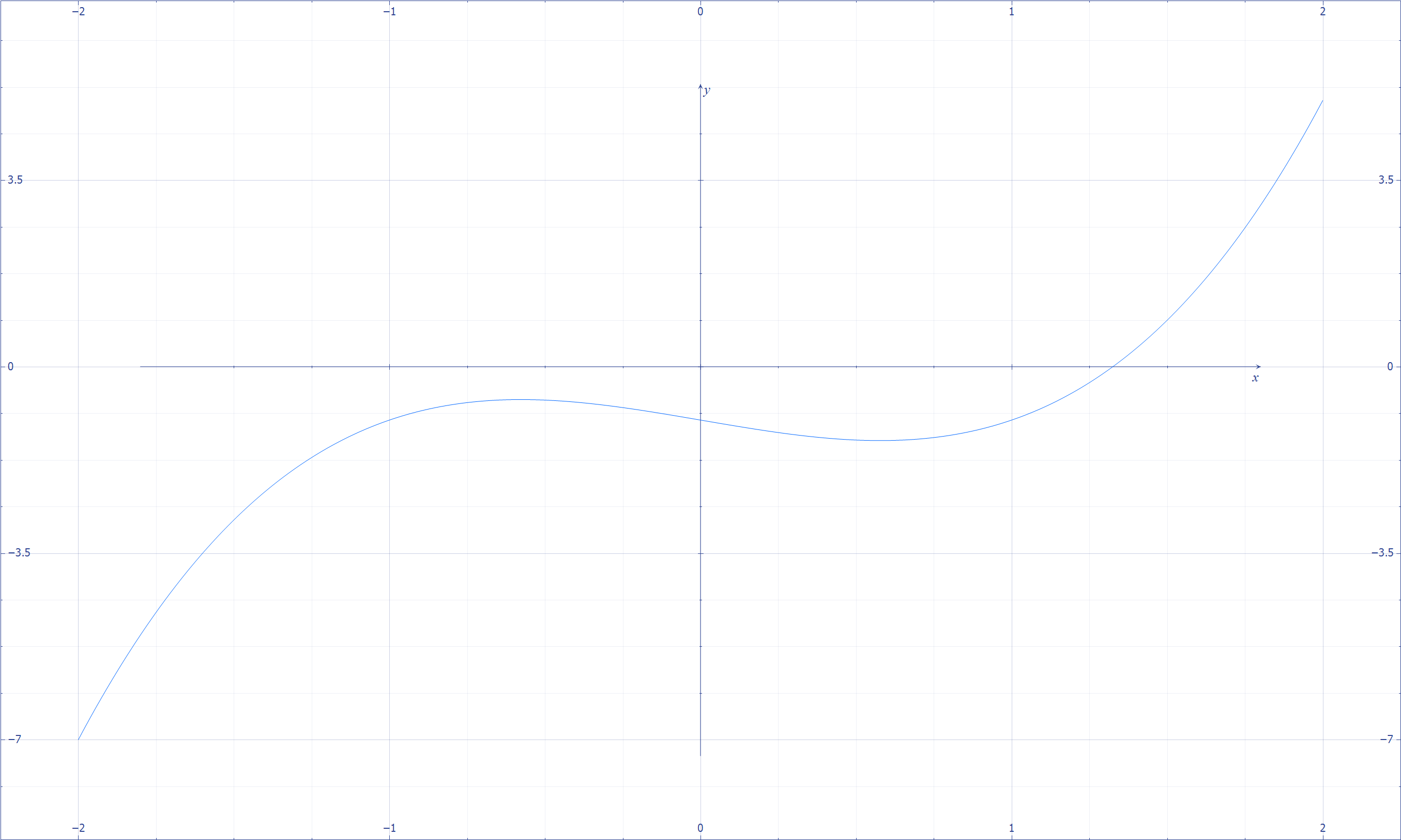# Graphing

Under Construction: Consider this content a preview of the real thing which is coming soon (hopefully).

graph LR
classDef currentPage stroke:#333,stroke-width:4px

ALG(["fas:fa-trophy Algorithmis fas:fa-trophy "])

ASY_ANA(["fas:fa-check Asymptotic Analysis#160;"])
click ASY_ANA "./math-asymptotic-analysis"

MAT_NOT(["fas:fa-check Mathematical Notation#160;"])
click MAT_NOT "./math-notation"

POL(["fas:fa-check Polynomials #160;"])
click POL "./math-polynomials"

MAT_FUN(["fas:fa-check Math Functions#160;"])
click MAT_FUN "./math-functions"

LOG(["fas:fa-check Logarithms#160;"])
click LOG "./math-logarithms"

COM(["fas:fa-check Combinatorics#160;"])
click COM "./math-combinatorics"

SET_NOT(["fas:fa-check Set Notation#160;"])
click SET_NOT "./math-set-notation"

GRA(["fas:fa-check Graphing#160;"])
click GRA "./math-graphing"

class GRA currentPage

ASY_ANA-->ALG
COM & GRA & SET_NOT-->ASY_ANA
MAT_NOT--> SET_NOT
POL & LOG--> MAT_FUN
MAT_FUN--> GRA


A function is representable as a curve in space where the vertical direction symbolizes the output and the horizontal direction symbolizes the input. The connection between polynomials as geometric objects and their algebraic properties is profound.Descartes graphical representation of polynomials